Next: What are numbers? Up: Algebraic structures Previous: Fields

## Ordering

Given a ring, we can say that it is ordered when you have a special subset of that ring behaves in a very special way. If any two elements of that special subset are added or multiplied their sum and their product are again in the special subset. Take the negative numbers in R, can they be that special subset? Well the sum seems to be allright, it is also a negative number. But things don't work with the product: it is positive. What about the positive numbers? Yep, and in fact we call that special subset, the set of positive elements. Now, we gave the definition for an ordered ring, we can also define an ordered field the same way.

But what does a complete ordered field mean? Well the definition looks rather nasty: it is complete if every non-empty subset which posesses an upper bound has a least upper bound.

Let's translate some of that, trying to lose as little information on the way. A bound is something that guarantees that all of the elements of your set are on one side of it (reasonably enough). For example, certainly all negative reals are less than 100, so 100 is a bound (it is in fact an upper bound 'cause all negatives are ``below'' it). But there are lots of other bounds, 1, 5, 26 will all do nicely. The question now is, of all of these (upper bounds) which is the smallest, that is which one is ``the border'' so to speak? Does it always exists?

Let's take the rationals, and look at the following numbers: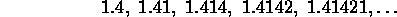Now each of these is a rational number (it can be written as a fraction), and they are getting closer and closer to a number we've probably seen before (just take out your calculator and find the square root of two). So we can write the shorthand for this series as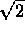. Certainly we can find an upper bound for this series, 3 will do nicely, but so can 1.5, or 1.42. But what is the smallest. Well there isn't any. Not among the rational at least, because no matter what fraction you give I can give you one closer to the square root of two. What about the square root of two itself? Well it's not a rational number (I'll skip the proof, but it is really rather easy) so you can't use it. If you want another series which is really neat look at the section on ``Euler's formula'' in the FAQ.

And that is where the reals come in. Any set or reals that is bounded you can certainly find the smallest of these bounds. (By the way this ``least upper bound'' is abbreviated ``l.u.b.'', or ``sup'' for supremum.) We can also turn things around and talk of lower bounds, and of the largest of these etc. but most of that will be just a mirror image of what we have dealt with so far.

So that should be it. And for years that did seem to be it, we seemed to have all the numbers we'd ever care to have.

There was just one small stick in the works, but most people just sort of pretended not to notice, and that was that not all polynomials had solutions. One simple polynomial of this kind is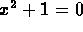. It's so simple, yet there's no self respecting number that would solve this polynomial. There were these funny answers which seemed like they should be solutions but no one could make any sense out of them, so they were considered imaginary solutions. Which was really too bad because they were given the name of imaginary numbers and now that the name stuck we realize that they are numbers just as good as any of the ones we have been using for centuries. And in fact that takes us to the last great pinnacle in this short excursion. The field of complex numbers.

We can define an algebraically closed field as a field where every nonconstant polynomial (i.e. one with an x in it from high school days) has a zero in the field. Whew! This in short means that as long as the polynomial is not a constant number (which is no fun anyways) but something which looks like it wants a solution, like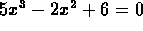it will always have one, if you are working with complex numbers and not just reals.

There is another definition which is probably just as good, but may or may not be easier: A field is algebraically closed if every polynomial splits into linear factors. Linear factors are briefly factors not containing x to any power of two or higher, in other words in the form: ax + b. For example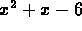can be factored as (x + 3)(x - 2), but if we are in the field of reals we cannot factor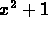, but we can in the field of complex numbers: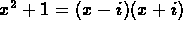, where, you may recall,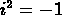.Next: What are numbers? Up: Algebraic structures Previous: Fields

Alex Lopez-Ortiz
Mon Feb 23 16:26:48 EST 1998